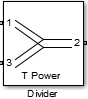# Divider

Model ideal frequency-independent dividers (combiners) with S-parameters

• Library:
• RF Blockset / Circuit Envelope / Junctions## Description

The Divider block models power dividers (combiners) in a circuit envelope environment as an ideal s-parameter model.

## Parameters

expand all

Divider type, specified as:

• `T power divider`

The s-parameter matrix for `T-power divider ` is :

$\left[\begin{array}{ccc}{s}_{11}& {s}_{21}& {s}_{31}\\ {s}_{21}& {s}_{22}& {s}_{32}\\ {s}_{31}& {s}_{32}& {s}_{33}\end{array}\right]$

where:

• s11 = (z23 –z1) / (z23 + z1)

• s22 = (z13 –z2) / (z13 + z2)

• s33 = (z12 –z3) / (z12 + z3)

• s21 = (1+ s11) * sqrt(z1 / z2)

• s31 = (1+ s11) * sqrt(z1 / z3)

• s32 = (1+ s22) * sqrt(z2 / z3)

• z12 = z1 * z2 / (z1 + z2)

• z13 = z1 * z3 / (z1 + z3)

• z23 = z2 * z3 / (z2 + z3)

• z1 = Z0(1), z2 = Z0(2), z3 = Z0(3)

• Reference Impedances: Z0 = [z1, z2, z3 ]

• `Resistive power divider`

The s-parameter matrix for `Resistive power divider` is :

$\left[\begin{array}{ccc}0& 1/2& 1/2\\ 1/2& 0& 1/2\\ 1/2& 1/2& 0\end{array}\right]$

When you select this option, the following parameter is available:

• `Wilkinson power divider`

The s-parameter matrix for `Wilkinson power divider` is:

$\left[\begin{array}{ccc}0& -j/\sqrt{2}& -j/\sqrt{2}\\ -j/\sqrt{2}& 0& 0\\ -j/\sqrt{2}& 0& 0\end{array}\right]$

### Note

For DC carrier (0 Hz) frequency, Wilkinson power divider is a zero matrix.

• `Tee H-plane (S33=0)`

The `Tee H-plane (S33=0)` is symmetrical, lossless, and reciprocal. The s-parameter matrix is:• `Tee E-plane`

The `Tee E-plane` is symmetrical, lossless, and reciprocal. The s-parameter matrix is:Reference impedance of divider, specified as a positive scalar or three-tuple vector.

Select this parameter to ground and hide the negative terminals. To expose the negative terminals, clear the parameter. By exposing these terminals, you can connect them to other parts of your model.

By default, this option is selected.